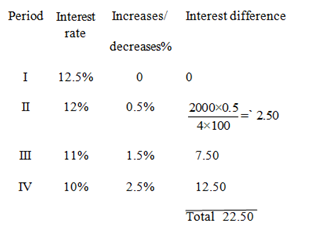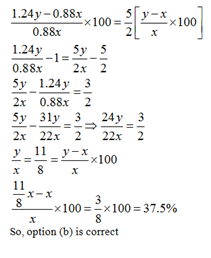# SSC Quantitative Aptitude Practice Questions (Day-3)

Dear Aspirants, Here we have given the Important SSC CGL Exams 2018 Practice Test Papers. Candidates, those who are preparing for SSC CGL Exams 2018 can practice these questions to get more confidence to Crack SSC CGL Exams 2018 Examination.

[WpProQuiz 4033]

1) If a2/5 = P, then the value of 2a1/6 – 3a2/15 in term of P will be

a) P 2/15 – 3P2/3

b) 2P 5/12 – 3P3/2

c) 2P 5/2 – 3P3/12

d) None of these

2) If x > 0, then which one is true?

a) x + 1/x ≤ 2

b) x + 1/x ≥ 2

c) x – 1/x ≤ 2

d) None of these

3) (sin 7θ– sin 5 θ)/(cos 7θ – sin 5θ) = x. Find the value of x.

a) Sin θ

b) Tan θ

c) Cos θ

d) Cot θ

4) Three boys are standing on a circular boundary of a fountain. They are at an equal distance from each other. If the radius of the boundary is 5m, the shortest distance between any two boys is

a) 5√3/2 m

b) 5√3

c) 15√3/2

d) 10π/3

5)  If a1/p = b1/q = c1/r and abc = 1, then the value of  p + q + r is equal to

a) 1

b) 0

c) 1/2

d) 3/2

6) Starting from the same point at the same time on a circular track 1248m long. Arvind moves in the clockwise direction and Ritesh and Dipesh move in anticlockwise. Amit meets Ritesh every 1 min and 18 sec and Amit meets Dipesh every 1 min 36 s. After every how many seconds does Ritesh meet Dipesh?

a) 12 s

b) 145 s

c) 416 s

d) 418 s

7) Which of the following can be the value of n for which (n11 – n) (92n – 1) is divisible by 164?

a) 27

b) 81

c) 40

d) 43

8) A cone circumscribes a sphere and its height is double the diameter of the sphere. The volume of the cone is K times the volume of the sphere. Then K is equal to

a) 3

b) 4

c) 2

d) 6

9) P is a 2 digit number ‘ab’, Q is a 3-digit number ‘cde’, if you multiply P and Q then multiply it by 9, then you will get abcde. What is the sum of P and Q?

a) 110

b) 104

c) 112

d) 126

10) A man borrows Rs.1000 and agrees to repay it with a total interest of Rs.140 in 12 installments, each installment less than the one preceding it by Rs.10. What should be his first installment? (Consider interest being considered at simple interest rate)

a) 100

b) 150

c) 200

d) 175

11) A can do a job in 36 days, B is 20% more efficient than A and C is 50% more efficient than B, B and C together can do the job in

a) 10 days

b) 12 days

c) 15 days

d) 8 days

12) In a class of 51 students, the difference between the highest marks and the least marks is 70. If the average score is calculated without considering the student who got the highest marks then the average score decreases by 1%. If the average score is calculated without considering the student who got the least marks then the average score increases by 1 1/3 %. What is the original average score of the class?

a) 60

b) 70

c) 80

d) 40

13) A shopkeeper mixes two varieties of sugar M and N in the ratio 2 : 5 and 3 : 7 so as to make two mixtures X1 and X2 respectively. He now mixes X1 and X2 in the ratio 1 : 4 and sells the compound mixture at Rs.15 per kg making a profit of 25%. Find the price of M (dearer variety) if price of N is Rs.10 per kg.

a) Rs 12.2 per kg

b) Rs 16.73 per kg

c) Rs 17.7 per kg

d) Rs 18.3 per kg

14) Seema invests Rs 2000 in 3 months fixed deposit scheme of HDFC Bank at a rate of interest of 12.5%. Due to some changes in government policies, the rate of interest changed in every three months, after the first period, to 12%, 11% and 10% respectively. Assuming that Seema with draws the interest after every 3 months but continues deposit, how much more interest would Seema has earned?

a) Rs 12.75

b) Rs17.50

c) Rs 20

d) Rs22.50

15) Ram sold an article at a certain profit. Had he purchased the article for 12% less than what he actually purchased it for and sold it for 24% more than what he actually sold it for, his profit percentage would have been 2 1/ 2 times his actual profit percentage? Find his actual profit percentage.

a) 27 ½ %

b) 28 2/7 %

c) 33 1/3 %

d) 37 ½ %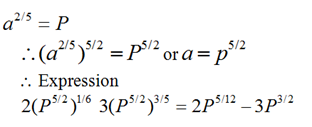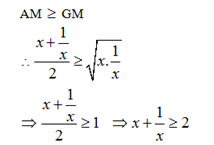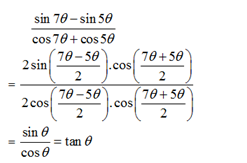Circumference of Circle ABC= πd = π10 m

AB = BC = CA = 10 π / 3 m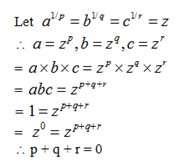Let their speeds be A m/s, C m/s and D m/s respectively for Arvind, Ritesh and Dipesh.

According to the question,

1248/ (A + C) = 78 or A + C = 16 — (i)

1248 / (A+D) = 96 or A + D = 13 — (ii)

Solving Eq (i) and (ii)

C – D = 3

Every second Ritesh gains 3 m over Dipesh. As they are moving in the same direction.

∴ Time taken by Ritesh to meet Dipesh.

= 1248/3 = 416 s

P = (n11 – n) (92n – 1) = (n11 –n)(81n – 1)

(n11 – n)[(82 – 1)n  −1]

For every integer (81n – 1) will be even i. e, a multiple of 2 and [(82 – 1)n – 1] is divisible by 82 only when n is even.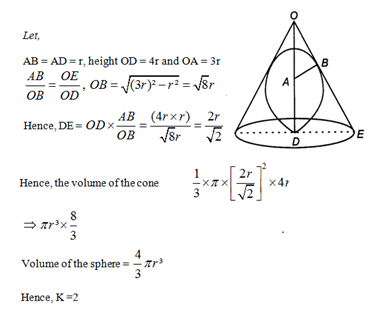Let number = abcde

= 1000 (ab) + cde

N = 1000P = Q = 9.P.Q

N = F (P, Q)

9PQ = 1000 + P + Q

If Q = 1000 then,

900 P < 1000 P + 100

P must be greater than 111

9 P.111= 999 P = 1000 P + Q

Check the options

9 × 112 × P = 1000P = 112

1008P = 1000P + 112

8P = 112   P = 14

P = 14, Q = 112 satisfy

9 PQ = 1000 P + Q

So, P + Q = 126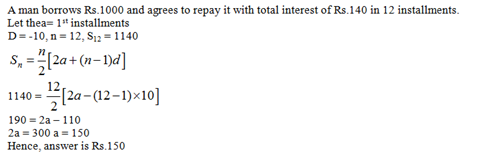Ratio of efficiency of A:B:C = 100:120:180 = 5 : 6 : 9

Efficiency of A is 5=36, therefore 1=36×5,

so B+C(6+9=15), 15= 36 ×5/15=12 Days

Suppose the average marks be x. Highest and least marks be p and q respectively,

Then, 51x = 50x × 0.99 + a

51x = 50 × 1.013 + b

50x × 0.99 + a = 50x × 1.013 + 6

a – b = 70[given]

70 = 50x [0.023]

x = 60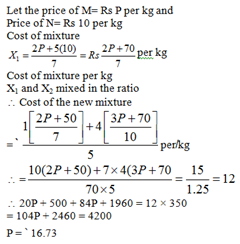The usual procedure adopted would be to compute the total interest earned for one year at 12.5% interest, the interest earned with the prevailing rates of interest and determine the difference. A faster way would be to compute only the increase or decrease in the interest earned in every period over 12.5%.